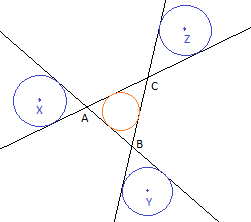# Outside Fun

Geometry Level 5The three blue circles with centers $X, Y$ and $Z$ are each tangent to two of the lines making up the triangle $ABC$ and each of them has twice the area of the incircle of the triangle $ABC$. If the area of the triangle $ABC$ is 1, find the area of the triangle $XYZ$ and express it as $a+b\sqrt{c}$, where $a,b$ and $c$ are positive integers with $c \neq 1$ square-free. Submit your answer as $a+b+c$.

×

Problem Loading...

Note Loading...

Set Loading...